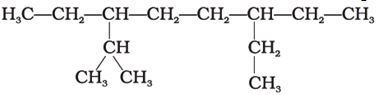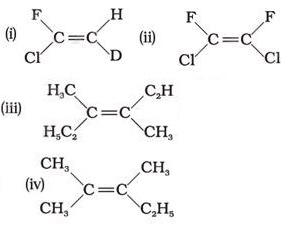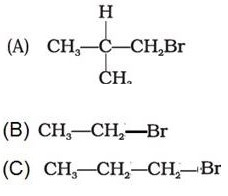# Topic: Hydrocarbons (Test 1)

Topic: Hydrocarbons
Q.1
In Friedel-Crafts alkylation reaction, when benzene is treated with an alkyl halide in the presence of a catalyst, alkylbenene is formed. The catalyst used is
A. anhydrous aluminium chloride
B.

C.
Silver
D.

Explaination / Solution:

the acid chloride or anhydride reacts with anhydrous aluminium chloride to form the acylium ion which serves as an electrophile which then attacks the benzene ring to give alkylbenzene.

Workspace
Report
Q.2
Some of the polynuclear hydrocarbons having fused benzene ring system have the property of being
A. malleable
B. nonflammable
C. conductive
D. carcinogenic
Explaination / Solution:

Polynuclear hydrocarbons are carcinogenic,i.e. cancer producing

Workspace
Report
Q.3
Arrange the following in decreasing order of their boiling points. (A) n–butane (B) 2–methylbutane (C) n-pentane (D) 2,2–dimethylpropane
A. A > B > C > D
B. D > C > B > A
C. C > B > D > A
D. B > C > D > A
Explaination / Solution:

Boiling point depends on molecular mass and surface area.As the number of carbon atoms increase,boiling point increases ,hence n-butane has the minimum boiling point.Among isomeric alkanes,boiling point decreases with branching.Hence the order.

Workspace
Report
Q.4

Arrange the halogens in order of their increasing reactivity with alkanes.

A. Br2< Cl2< F2< I2
B. Br2< I2< Cl2< F2
C. I
2
< Br2< Cl2< F2
D. F2< Cl2< Br2< I2
Explaination / Solution:

The reaction follows free radical mechanism in which the first step is generation of halogen free radical which is the fastest for fluorine.Hence the order.

Workspace
Report
Q.5
The increasing order of reduction of alkyl halides with zinc and dilute HCl is
A. R–Cl < R–I < R–Br
B. R–Br < R–I < R–Cl
C. R–Cl < R–Br < R–I
D. R–I < R–Br < R–Cl
Explaination / Solution:

As we move from I to Br to Cl,atomic size decreases,it becomes difficult to release the halogen as the bond length decreases.Hence I- is the best leaving group among halogens and reacts the fastest.

Workspace
Report
Q.6
The correct IUPAC name of the following alkane isA. 3,6 – Diethyl – 2 – methyloctane
B. 5 – Isopropyl – 3 – ethyloctane
C. 3 – Isopropyl – 6 – ethyloctane
D. 3 – Ethyl – 5 – isopropyloctane
Explaination / Solution:

Following the rules of nomenclature,the IUPAC name of the given compound is 3,6-Diethyl-2-methyloctane

Workspace
Report
Q.7
Which of the following will not show geometrical isomerism?A. (ii)
B. (iii)
C. (iv)
D. (i)
Explaination / Solution:

This is because geometrical isomerism is not possible if three groups are same.

Workspace
Report
Q.8
Arrange the following hydrogen halides in order of their decreasing reactivity with propene.
A. HBr > HI > HCl
B. HI > HBr > HCl
C. HCl > HBr > HI
D. HCl > HI > HBr
Explaination / Solution:

As we move from Cl to I,the atomic size increases,bond length increases,bond strength decreases,hence it becomes easier to release halogen and hence the order.I- is the a better leaving group.

Workspace
Report
Q.9
Arrange the following carbanions in order of their decreasing stability.(A) H3C – C $\equiv$C (B) H – C ≡ C (C)
A. C > A > B
B. A > B > C
C. C > B > A
D. B > A > C
Explaination / Solution:

In B,the '-' charge is on sp hybridised C atom so the negative charge is stabilised.In A,there is an alkyl group attached to sp hybridised carbon which destabilises the negative charge. In C,the '-' charge is on a sp3 hybridised carbon and is hence the least stable.

Workspace
Report
Q.10
Arrange the following alkyl halides in decreasing order of the rate of  elimination reaction with alcoholic KOHA. C > B > A
B. A > C > B
C. B > C > A
D. A > B > C